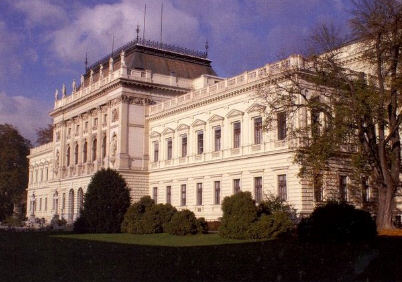# Invariants of 2nd Order: Iterated IPADsGoing Beyond Current Limits:

• We just invented the concept of Iterated IPADs.
This is a revolutionary new method
of filtering possible p-tower groups
by computing Invariants of Second Order:

• Iterated IPAD Sweeps 2-Tower GroupsThe New Method: ************************************************************************* For each unramified cyclic extension L|K (of 1st order) of degree p over a given base field K, we additionaly determine its unramified cyclic degree p extensions N|L of 2nd order. Consequently, we end up getting an iterated or extended IPAD (index-p abelianization data) τp2(K), that is a family of IPADs [τ(K);τ(L1);...;τ(Ln)] with suitable n. (We avoid the terminology "extended TTT", since we do not know yet whether these new abelianizations are associated to transfers.) ************************************************************************* We briefly explain our ideas by three examples, the first two in connection with three-stage towers of 2-class fields. ----------------------------------------------------------------------- Example 1. p = 2, K = Q((-1780)1/2) with Cl2(K) ~ (2,4) has n = 3 and τ22(K) = [τ(K);τ(L1);...;τ(L3)] = [(2,2,2),(4,4),(2,8); (2,2,4)6,(4,4); (2,2,4)3; (2,2,4),(2,16)2], as mentioned and used in M. R. Bush's 2003 paper . Of course, the extension K((13+4*51/2)1/2) cannot be cyclic or abelian over K. It must be non-Galois! ----------------------------------------------------------------------- Example 2. p = 2, K = Q((-2067)1/2) with Cl2(K) ~ (2,4) has n = 3 and τ22(K) = [τ(K);τ(L1);...;τ(L3)] = [(2,2,2),(4,4),(2,8); (2,2,2,2)3,(2,2,8)2,(2,2,4),(4,4); (2,2,8)2,(2,2,4); (2,2,4),(4,8)2]. ----------------------------------------------------------------------- And now some hopeful expectations, shedding light on the infinite family by L. Bartholdi and M. R. Bush, 2007 : Example 3. p = 3, K = Q((-3896)1/2) with Cl3(K) ~ (3,3) has n = 4 and τ32(K) = [τ(K);τ(L1);...;τ(L4)] = [(3,3,3),(3,9),(3,3,3),(3,3,3); (3,3,9)4,(3,3)9; (3,3,9),(3,27)3; (3,3,9)4,(9,9)9; (3,3,9)4,(9,9)9]. For these fields of absolute degree 18, Magma has to struggle considerably, but it finally succeeds. Similar results were obtained for the discriminants d = �6583,-23428,-25447,-27355,-27991,-36276,-37219,-37540. -------------------------------------------------------------- We should now give an exact definition of the iterated IPAD and emphasize that its interpretation via transfers seems to be problematic. We cannot see how to express the components of second order by means of any higher p-class group Gal(Fps(K)|K), s > 1, of a given base field K. -------------------------------------------------------------- Definition. Let p be a prime, r > 1 an integer, K|Q a number field with p-class group Clp(K) of rank r, m = (pr-1)/(p-1), and (L1,...,Lm) the family of unramified cyclic extensions of K of relative degree p and absolute degree p*[K:Q]. For each i in {1,...,m}, let ri be the p-class rank of Li, ni = (p^{ri}-1)/(p-1), and (Ni,j)_{j in {1,...,ni}} the family of unramified cyclic extensions of Li of relative degree p and absolute degree p2*[K:Q]. The iterated IPAD τp2(K) of K consists of a single component of first order, [Clp(Li),...,Clp(Lm)], and m components of second order, [Clp(Ni,j)]_{j in {1,...,ni}} for i in {1,...,m}. -------------------------------------------------------------- Remark 1. For p = 2 and a base field K of type (2,4), the iterated IPAD contains the second layer of the TTT τ(K) of K, since m = 3 and there exists i in {1,...,3}, such that Li is common subfield of the 3 unramified extensions of relative degree 4 of K. -------------------------------------------------------------- Remark 2. For p = 3 and a base field K of type (3,3), each component of second order of τ32(K) includes the abelian type invariants of the Hilbert 3-class field of K, since m = 4 and for each i in {1,...,4} there exists j in {1,...,ni}, such that Ni,j = F31(K). -------------------------------------------------------------- While there is no difficulty to form composita of class extension homomorphisms: J(Li|K) : Clp(K) → Clp(Li) and J(Ni,j|Li) : Clp(Li) → Clp(Ni,j) yield together J(Ni,j|Li) * J(Li|K) = = J(Ni,j|K) : Clp(K) → Clp(Ni,j), the corresponding transfers from the p-groups G = Gal(Fps(K)|K), s > 1, resp. Gi = Gal(Fpt(Li)|Li), t > 1, to their subgroups Hi = Gal(Fps(K)|Li), resp. Hi,j = Gal(Fpt(Li)|Ni,j), T(G,Hi) : G/G' → Hi/Hi' and T(Gi,Hi,j) : Gi/Gi' → Hi,j/Hi,j' cannot be concatenated immediately. An isomorphism f : Hi/Hi' ~ Gal(Fp1(Li)|Li) ~ Gi/Gi' must be inserted to obtain a formal compositum T(G_i,Hi,j) * f * T(G,Hi) = T(G,Hi,j) : G/G' → Hi,j/Hi,j' and we have doubts whether this formal compositum can be viewed as a transfer, since Hi,j is not a subgroup of G, and whether it can be associated to J(Ni,j|K), in general with non-abelian extension Ni,j|K. --------------------------------------------------------------Some Caveats:

• It seems that this non-abelian problem
must be tackled from the
viewpoint of projective limits,
namely the p-tower group
Gal(Fp(K)|K)
of the maximal unramified
pro-p extension of K.

• Finally it should be remarked that
we are trying to identify
G = Gal(Fps(K)|K) via τp2(K),
but Gi = Gal(Fpt(Li)|Li) is
(and will probably remain)
completely unknown.

 * Web master's e-mail address: contact@algebra.at *